Students can download 5th Maths Term 2 Chapter 2 Numbers InText Questions and Answers, Notes, Samacheer Kalvi 5th Maths Guide Pdf helps you to revise the complete Tamilnadu State Board New Syllabus, helps students complete homework assignments and to score high marks in board exams.

## Tamilnadu Samacheer Kalvi 5th Maths Solutions Term 2 Chapter 2 Numbers InText Questions

Try These (Text Book Page No. 5)

12 = 1 × 1 = 1
22 = 2 × 2 = 4
32 = 3 × 3 = 9
42 = 4 × 4 = 16
52 = 5 × 5 = 25
62 = 6 × 6 = ______
72 = 7 × 7 = ______
82 = 8 × 8 = ______
92 = 9 × 9 = ______
102 = 10 × 10 = ______
12 = 1 × 1 = 1
22 = 2 × 2 = 4
32 = 3 × 3 = 9
42 = 4 × 4 = 16
52 = 5 × 5 = 25
62 = 6 × 6 = 36
72 = 7 × 7 = 49
82 = 8 × 8 = 64
92 = 9 × 9 = 81
102 = 10 × 10 = 100Activity (Text Book Page No. 6)

A. Count and Write the number of boxes:

Question 1.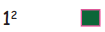1

Question 2.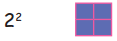4

Question 3.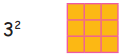9

Question 4.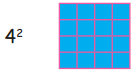16B. Circle and colour the square numbers:

Question 1.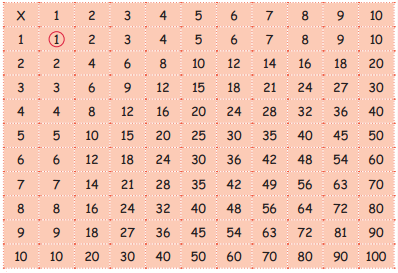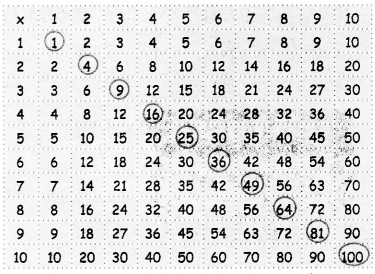Work Sheet (Text Book Page No. 7)

Question 1.
The square number of 2 is _________
Hint : 2 × 2 = 4
4

Question 2.
The square number of 5 is
hint : 5 × 5 = 25
25

Question 3.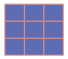These number of boxes is equal to one square number,
The number is ________
The number is 9Question 4.
Which of following number is square number
a. 23
b. 54
c. 36
d. 45
HInt = 62 = 6 × 6 = 36
36

Question 5.
What is next square number of 49?
a. 76
b. 95
C. 64
d. 45
Hint : 7 × 7 = 49
8 × 8 = 64
64Try These (Text Book Page No. 8)

Find the Factors:
Factors of 4 are 1, 2 and 4.
Factors of 10 are 1, 2, 5 and 10.
Factors of 16 are 1, 2, 4, 8 and 16.
Factors of 18 are ________
Factors of 20 are ________
Factors of 24 are ________
Factors of 42 are ________
Factors of 18 are ________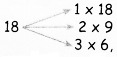1,2,3,6,9 and 18 are the factors of 18.

Factors of 20 are ________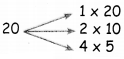1,2,4,5,10 and 20 are factors of 20.

Factors of 24 are ________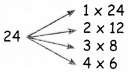1,2,3,46,8, 12 and 24 are factors of 24.

Factors of 42 are ________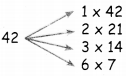1,2,3,6,7,14,21 and 42 are factors of 42.Activity (Text Book Page No. 9)

Tick the factors of the following numbers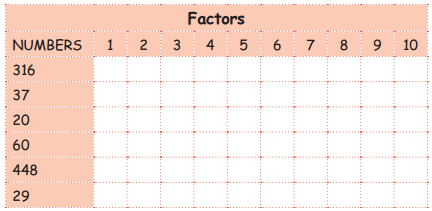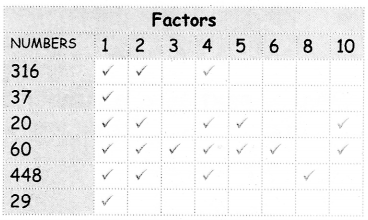Try These (Text Book Page No. 11)

5, 10, 15, _____, _____, _____, _____
20, 25, 30, 35, 40Try These (Text Book Page No. 12)

Find L.C.M
1. 10 and 15
2. 8 and 6
3. 4 and 10
4. 6 and 16
1. 10 and 15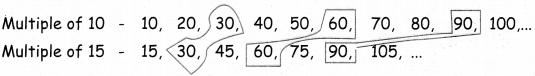Common multiples of 10, 15 are 30, 60, 90, …
LCM of 10, 15 is 30.

2. 8 and 6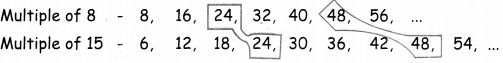Common multiples of 6, 8 are 24, 48,
LCM of 6, 8 is 24.3. 4 and 10
Multiple of 4 = 4, 8, 12, 16, 20, 24, 28, 32, 36, 40, 44, …
Multiple of 10 = 10, 20, 30. 40, 50, 60, …
Common multiples of 4, 10 ore 20, 40, …
LCM of 4, 10 is 20.

(4) 6 and 16
Multiple of 6 – 6, 12, 18, 24, 30, 36, 42, 48, 54, 60, 66, 72, 78, 84, 90, 96, 102, …
Multiple of 16 – 16, 32, 48, 64, 80, 96, 112, …
Common multiples of 6. 16 are 48, 96, …
LCM of 6, 16 is 48.Think it (Text Book Page No. 12)

Can we say the highest common multiples for two numbers?